﻿ Combustion Analysis

#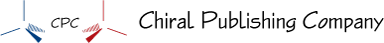Combustion Analysis

Obtaining Empirical and Molecular Formulas from Combustion Data

Empirical and molecular formulas for compounds that contain only carbon and hydrogen (CaHb) or carbon, hydrogen, and oxygen (CaHbOc) can be determined with a process called combustion analysis. The steps for this procedure are

Weigh a sample of the compound to be analyzed and place it in the apparatus shown in the image below.

Burn the compound completely. The only products of the combustion of a compound that contains only carbon and hydrogen (CaHb) or carbon, hydrogen, and oxygen (CaHbOc) are carbon dioxide and water.

The H2O and CO2 are drawn through two tubes. One tube contains a substance that absorbs water, and the other contains a substance that absorbs carbon dioxide. Weigh each of these tubes before and after the combustion. The increase in mass in the first tube is the mass of H2O that formed in the combustion, and the increase in mass for the second tube is the mass of CO2 formed.

Assume that all the carbon in the compound has been converted to CO2 and trapped in the second tube. Calculate the mass of carbon in the compound from the mass of carbon in the measured mass of CO2 formed.

Assume that all of the hydrogen in the compound has been converted to H2O and trapped in the first tube. Calculate the mass of hydrogen in the compound from the mass of hydrogen in the measured mass of water.

If the compound contains oxygen as well as carbon and hydrogen, calculate the mass of the oxygen by subtracting the mass of carbon and hydrogen from the total mass of the original sample of compound.

Use this data to determine the empirical and molecular formulas in the usual way.Apparatus for Combustion Analysis     A compound containing carbon and hydrogen (CaHb) or carbon, hydrogen, and oxygen (CaHbOc) is burned completely to form H2O and CO2. The products are drawn through two tubes. The first tube absorbs water, and the second tube absorbs carbon dioxide.

To illustrate how empirical and molecular formulas can be determined from data derived from combustion analysis, let’s consider a substance called trioxane. Formaldehyde, CH2O, is unstable as a pure gas, readily forming a mixture of a substance called trioxane and a polymer called paraformaldehyde. That is why formaldehyde is dissolved in a solvent, such as water, before it is sold and used. The molecular formula of trioxane, which contains carbon, hydrogen, and oxygen, can be determined using the data from two different experiments. In the first experiment, 17.471 g of trioxane is burned in the apparatus shown above, and 10.477 g H2O and 25.612 g CO2 are formed. In the second experiment, the molecular mass of trioxane is found to be 90.079.

We can get the molecular formula of a compound from its empirical formula and its molecular mass. (See the text for a reminder of how this is done.) To get the empirical formula, we need to determine the mass in grams of the carbon, hydrogen, and oxygen in 17.471 g of trioxane. Thus we need to perform these general steps.

First, convert from the data given to grams of carbon, hydrogen, and oxygen.

Second, determine the empirical formula from the grams of carbon, hydrogen, and oxygen.

Third, determine the molecular formula from the empirical formula and the given molecular mass.

Because we assume that all the carbon in trioxane has reacted to form in CO2, we can find the mass of carbon in 17.471 g trioxane by calculating the mass of carbon in 25.612 g CO2.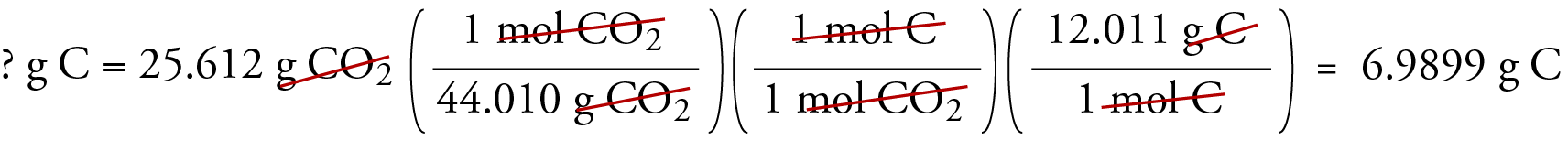Because we assume that all of the hydrogen in trioxane has reacted to form H2O, we can find the mass of hydrogen in 17.471 g trioxane by calculating the mass of hydrogen in 10.477 g H2O.Because trioxane contains only carbon, hydrogen, and oxygen, we can calculate the mass of oxygen by subtracting the masses of carbon and hydrogen from the total mass of trioxane.

? g O  =  17.471 g trioxane  - 6.9899 g C  -  1.1724 g H  =  9.309 g O

We now calculate the empirical formula, the empirical formula mass, and the molecular formula.Sample Study Sheet: Calculating Molecular Formulas from Combustion Data

Tip-off – You want to calculate the molecular formula of a compound containing only carbon and hydrogen (CaHb) or carbon, hydrogen, and oxygen (CaHbOc), and you are given its molecular mass and the masses of CO2 and H2O formed in the combustion of a given mass of the compound.

General Procedure

Calculate the number of grams of carbon in the compound by calculating the number of grams of carbon in the given amount of CO2.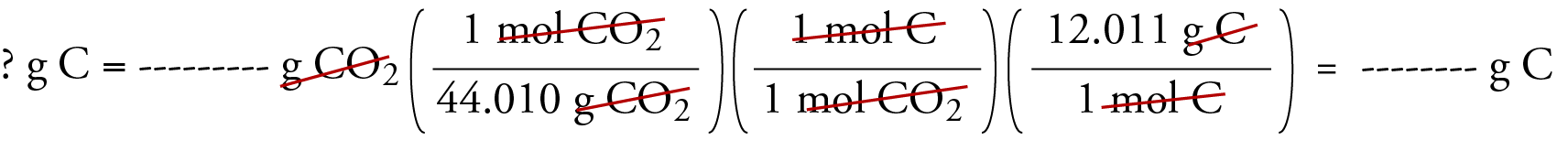Calculate the number of grams of hydrogen in the compound by calculating the number of grams of hydrogen in the given amount of H2O.If the compound contains oxygen, calculate the number of grams of oxygen in it by subtracting the masses of carbon and hydrogen from the given total mass of compound.

? g O  =  (given) g total  -  (calculated) g C  -  (calculated) g H

Calculate the empirical formula of the compound from the grams of carbon, hydrogen, and oxygen.

Calculate the formula mass for the empirical formula and divide the given molecular mass by the empirical formula mass to get n.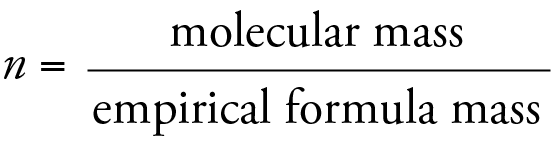Multiply each of the subscripts in the empirical formula by n to get the molecular formula.

EXAMPLE – Obtaining a Molecular Formula from Combustion Data:

Dianabol is one of the anabolic steroids that has been used by some athletes to increase the size and strength of their muscles. It is similar to the male hormone testosterone. Some studies indicate that the desired effects of the drug are minimal, and the side effects, which include sterility and increased risk of liver cancer and heart disease, keep most people from using it. The molecular formula of Dianabol, which consists of carbon, hydrogen, and oxygen, can be determined using the data from two different experiments. In the first experiment, 14.765 g of Dianabol is burned, and 43.257 g CO2 and 12.395 g H2O are formed. In the second experiment, the molecular mass of Dianabol is found to be 300.44.  What is the molecular formula for Dianabol?

Solution: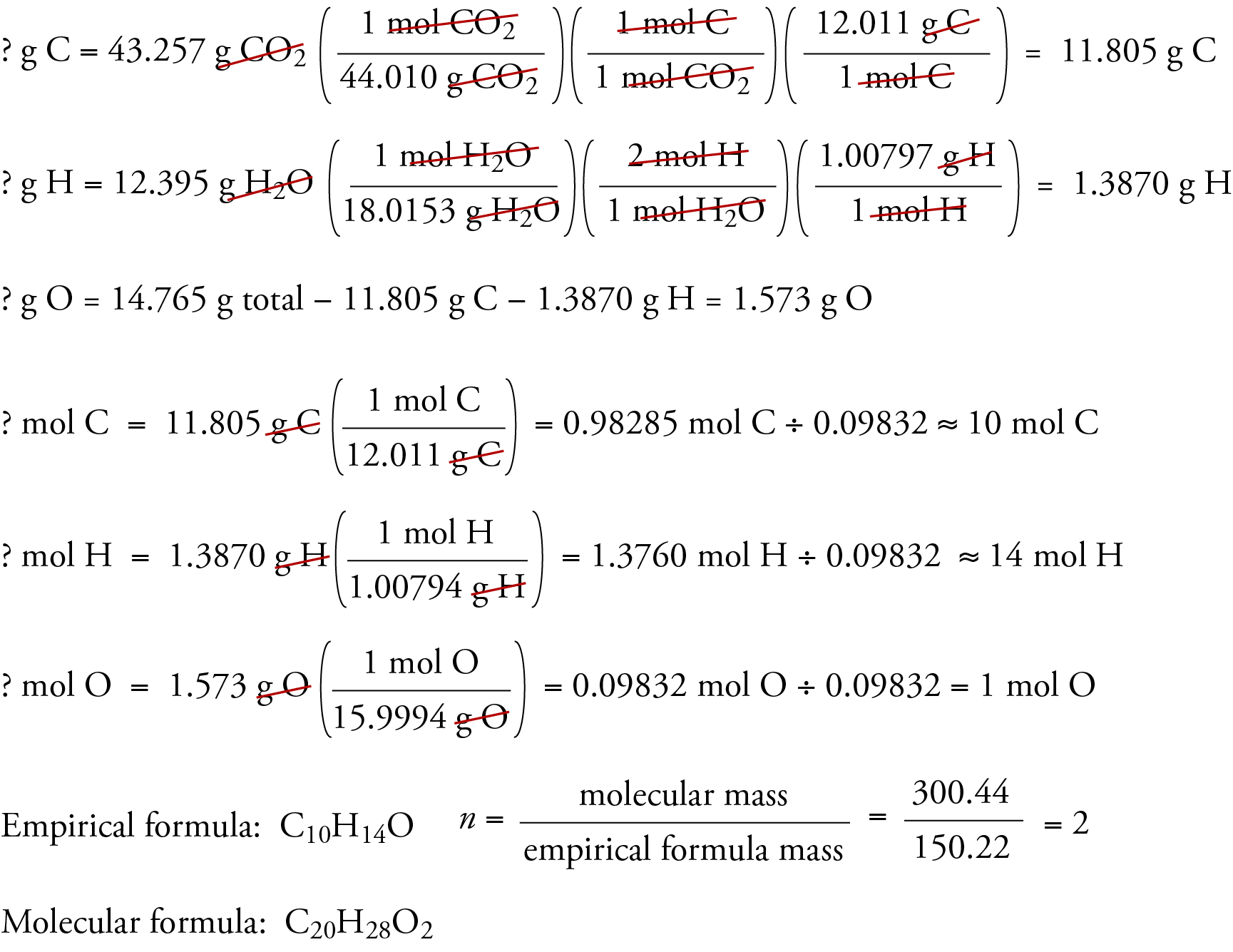Chiral Publishing Company